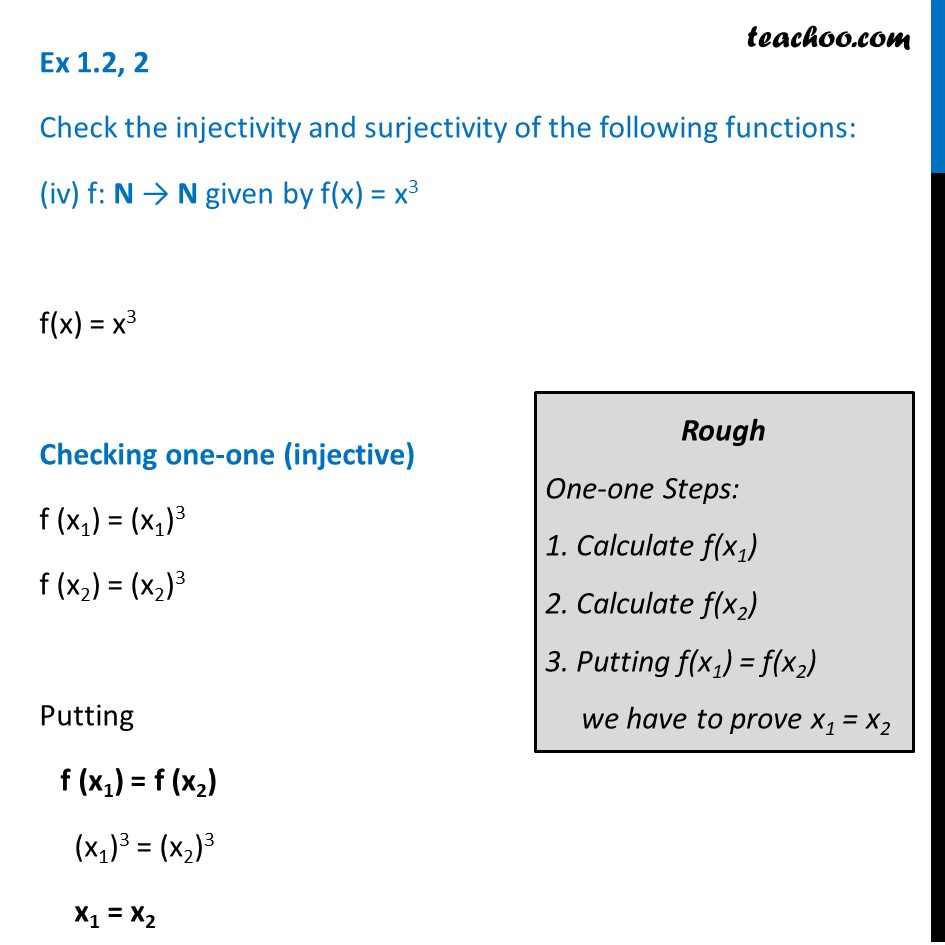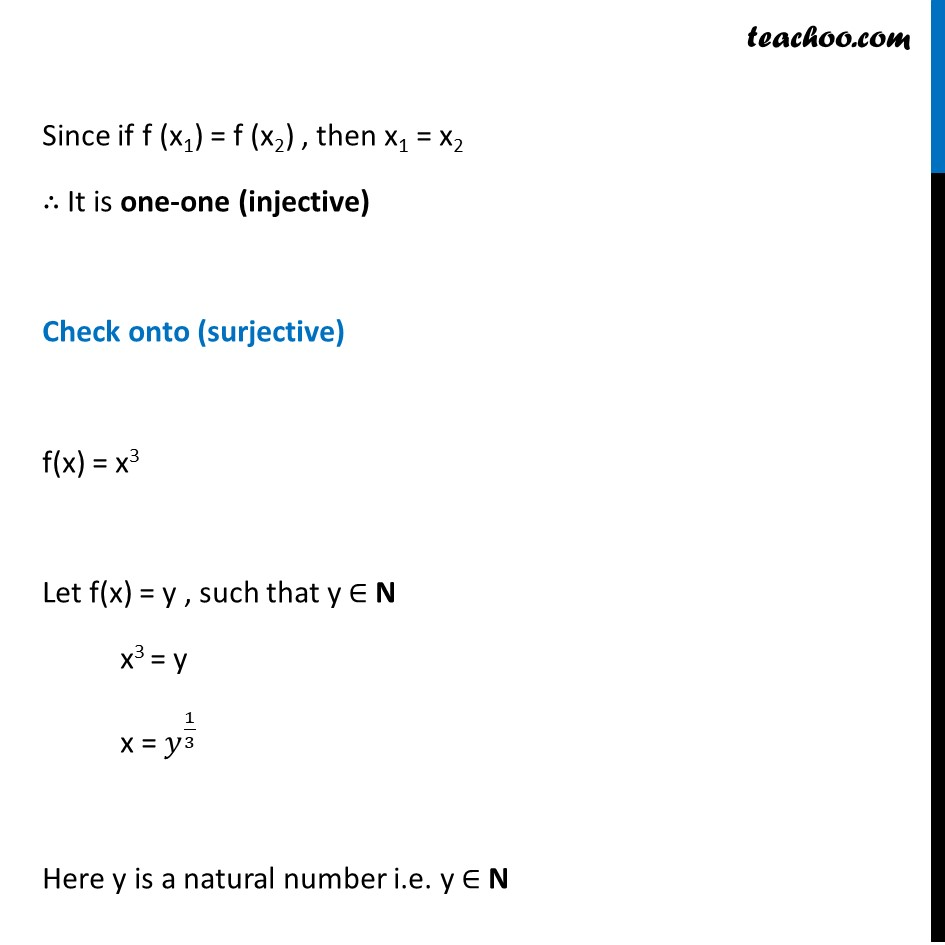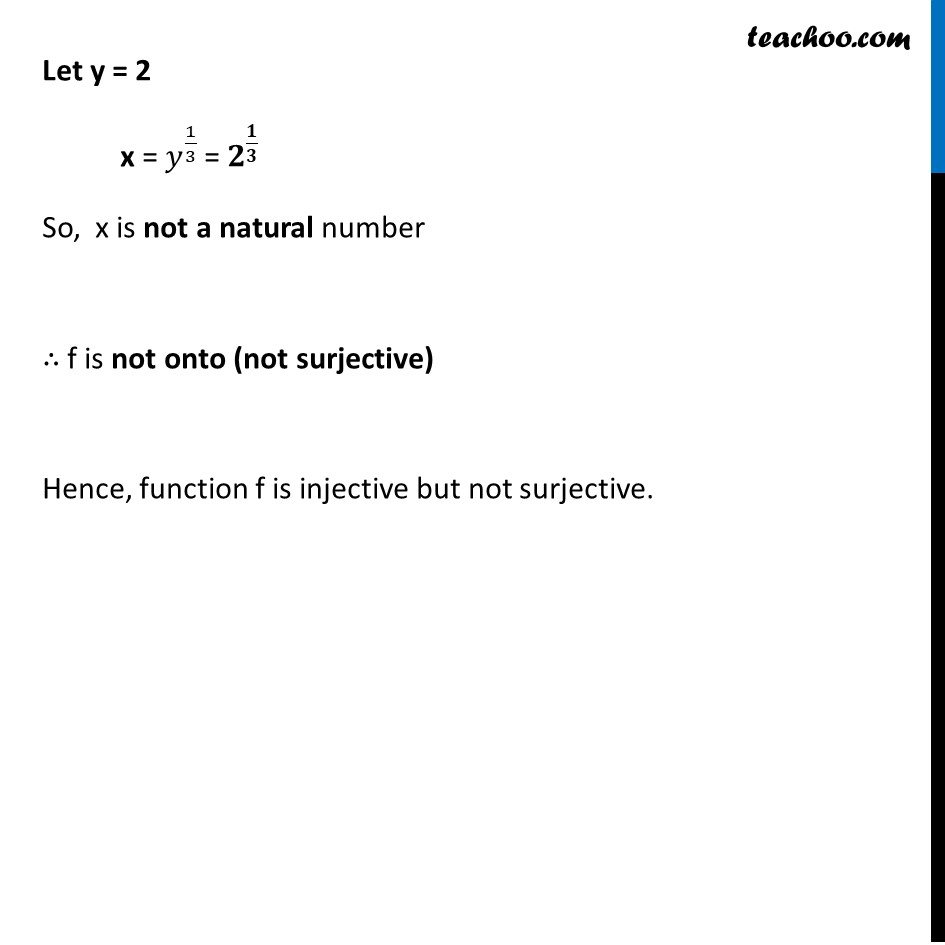Ex 1.2

Chapter 1 Class 12 Relation and Functions
Serial order wiseLearn in your speed, with individual attention - Teachoo Maths 1-on-1 Class

### Transcript

Ex 1.2, 2 Check the injectivity and surjectivity of the following functions: (iv) f: N → N given by f(x) = x3 f(x) = x3 Checking one-one (injective) f (x1) = (x1)3 f (x2) = (x2)3 Putting f (x1) = f (x2) (x1)3 = (x2)3 x1 = x2 Since if f (x1) = f (x2) , then x1 = x2 ∴ It is one-one (injective) Check onto (surjective) f(x) = x3 Let f(x) = y , such that y ∈ N x3 = y x = 𝑦^(1/3) Here y is a natural number i.e. y ∈ N Let y = 2 x = 𝑦^(1/3) = 𝟐^(𝟏/𝟑) So, x is not a natural number ∴ f is not onto (not surjective) Hence, function f is injective but not surjective.